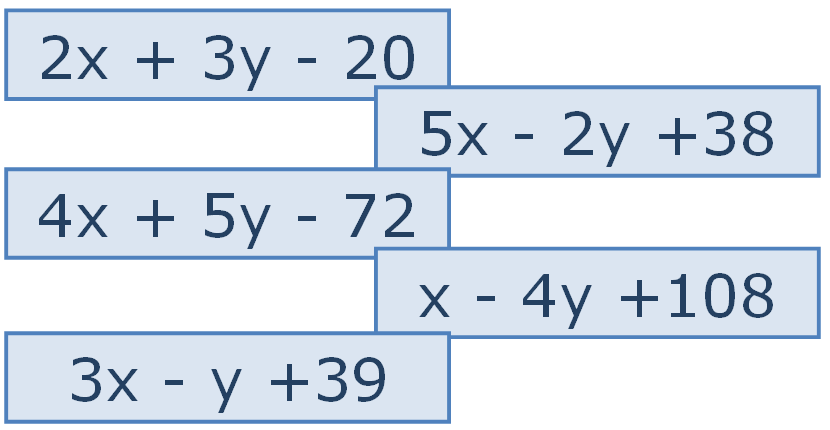Copyright © University of Cambridge. All rights reserved.

## 'Matchless' printed from https://nrich.maths.org/

### Show menu

There is a particular value of $x$, and a value of $y$ to go with it, which make all five expressions equal in value.
Can you find that $x$, $y$ pair?Did you have more information than you needed to solve the problem?